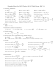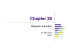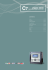# Applications of Magnetism

## Transcription

Applications of Magnetism
```PHY122 – Physics for the Life Sciences II
Lecture 15
ERLC AC-circuits
Review Chapters 24 - 26
Note: Clicker Channel 41
03/31/2015
Lecture 15
1
Chapter 26 – AC Electricity
• AC Voltage Sources
• AC Resistor circuits
– AC currents in a resistor
– AC power and RMS voltage and current
• AC Capacitor circuits
– AC voltage and current in a capacitor
– Reactance of a capacitor
• Inductors
– AC voltage and current in an inductor
– Self Inductance and Reactance
• Transformers
– Electrical Power in your home and Electrical safety
• AC circuits with R, C, and L
– ERLC circuits
03/31/2015
Lecture 15
2
Specific AC Circuits with EAC, R, C, and L
• We can now construct circuits with all passive
• and calculate (using Kirchhoff’s Rules!)
the currents and voltages …
i(t)
• We will only consider the simplest
circuits, consisting of a SERIES
connection of EAC, R, C, and L :
EAC
L
R C
• For this, we need to be able to add voltages TAKING
CARE OF THE PHASES of the voltages vR(t), vC(t) and
vL(t), with respect to the common current i(t) in the
circuit …
 Phasors!
03/31/2015
Lecture 15
3
Phasors…
Thus, the current i(t) (common to all elements in series) and
voltage v(t) have two aspects:
– an AMPLITUDE/MAGNITUDE I, V
– a time-dependent PHASE angle θ=ωt
I
θ=ωt
Phasor
i(t)=I cosωt
these two properties can be illustrated in a simple way by
representing the current and voltage as PHASORS:
– time-dependent vectors, rotating with constant angular velocity ω
• Note: i(t), v(t) are NOT vectors; phasors are just a graphic and useful tool to work
with time-dependent quantities of this sort!
in L:
in R:
in C:
V
in sync
Phasors
I
ωt
v(t)=
RIcosωt
03/31/2015
i(t)=
Icosωt
V phasor
of I phasor
I
v(t)=
–ωLI sinωt
Lecture 15
ωt
i(t)=
Icosωt
V phasor
90° behind
I phasor
v(t)=
(1/ωC)I sinωt
I
V
ωt
i(t)=
Icosωt
4
AC Circuits
i(t)
L
EAC
Series LRCEAC circuit, V vs. I:
R C
– Phasor diagram:
VL
V
φ
VR
v(t)
The total impedance Z:
I
ωt
i(t)
VL  VC 
V
V  VC  VR
Z
 L

I
I
 XL I  XC I    RI 
2

I
VC
2
 VR2
I
2

 XL  XC 
2
 R2
– the total voltage v(t) across L, C, and R (i.e. proper sum of VL, VC,
and VR, equal to VE, can be found by phasor addition, analog to
2
v(t )   L  1 C  I sin t  RI cos t   L  1 C   R 2 I cos t   
 
XL
XC
 Z I cos t    ;   arctan  L  1 C  R 
If L is absent: XL=0; if there is no capacitor: XC=0 …
03/31/2015
Lecture 15
5
Resonance
Let’s look at the current in a series ELRC circuit for a given
AC voltage (like the 120 V household power outlet):
v(t )  Z
I cos t    
  L  1 C 
2
 R 2 I cos t   ;   arctan  L  1 C  R 
V0
I
1
  
V0 Z
1/R1
1
  L  1 C   R 2
The I/V0 ratio is a function of ω,
and in fact, has a maximum
1
1/R for XL=XC: X L   L  X C 
 
C
2
I/V0
0
 0 
1
LC
1/R2,
R2>R1
0
ω0
ω
the NATURAL frequency !
The height of the resonance peak depends inversely on R …
At resonance: ω=ω0, XL=XC, and φ=0:
voltage and current are in sync at the resonance frequency!
Current: i(t)=v(t)/R; I=V/R, and Irms=Vrms/R !
03/31/2015
Lecture 15
6
The natural frequency ω0 of a series ELRC
series circuit with E=9.0 Vrms, L=2.0 H, and
R= 4.0 Ω, and C= 8.0 μF equals …
Responses
250
0.25
375
0.002
4
Other
0 
1
LC
82%
10%
6%
03/31/2015
Lecture 15
1%
4
00
2
1%
0.
37
5
0.
25
25
0
1%
Ot
he
r
Rank
1
2
3
4
5
6
7
Series LCR Circuit
Quantative discussion:
a
– Apply Kirchhoff #2
Note that i= –dq/dt: if q(t) decreases, i(t)>0, as sketched
2
di
q
d q
dq
VC  L  Ri  0   L 2  R  0
dt
C
dt
dt
2
d q
dq 1
damping term
L 2 R  q0
dt
dt C
t 
– Trial solution: q (t )  q0 e d cos t
– Then:
E

oscillating
term
S
b
C

i(t)
L
R
ONLY correct for
“underdamped” case
dq q
 q0 e t  d sint

dt

q 2
 t  d
d 2q q 
t  d
t  d
2


q
e
cos

t


q0 e t  d sin t   2 q


q
e
sin

t

q
e
sin

t
0
0
0
2
2
2


 d d
d d
d
dt
q (t )
– Note the appearance of sinωt and cosωt terms;
 must cancel independently  2 eq’ns:
sin t : L
03/31/2015
2
d
 R  0
 2L   d R   d  2L R
 R2
1
R2
R
1 R2 R2
1
1
2
2
2
cos t :
 2  02  2  02  d2
 L 2    R  0   L      
LC 4 L
2L
C 4L 2L
d
C  4L

Lecture 15
8
Discussion: LCR Circuits
Solution:
This is an exact analog to damped Simple
Harmonic Motion! Damping: ωd=b/(2m),
natural frequency: ω0=√(k/m)
q(t )  q0 e t  d cos t  q0 e d t cos t
R
1
2
2
,


 0
with   0  d , 0 
d
2L
LC
extreme case: R=0  ωd=0  NO damping , and ω2=ω02=1/LC …
– the quantity ω0 is the “natural frequency” of the system
– charge, current and (capacitor) voltage vs t:
qR 0 (t )  q0 cos 0t  q0 cos
 i (t )  
1
t
LC
dq
 0 q0 sin 0t  i0 sin 0t
dt
 vC (t ) 
Numerical example: R=1.0 Ω, L=20μH, C=500pF:
q
q
 0 cos 0t
C
C
2 L 40 μ  s
– The damping is small:  d 

 40 μs  d  25 103 s 1
R
1.0 
1
1
1
0 


 0  1.0 107 s 1
LC
20  106  500  1012
1.0 1014 s 2
– The circuit, once started, oscillates with frequency:
1
1

 d2 
 25  103 s 1  5 1013 s 1    7.1106 s 1
LC
40 μ  s  500 pF

 f 
 1.1MHz
2
03/31/2015
Lecture 15
9
A capacitor C, initially charged to q0, is connected
in series an inductor L.
What would happen to the period of oscillations T
and the maximum current Imax if the same charged
capacitor were connected to a larger inductance?
A.
B.
C.
D.
E.
F.
G.
H.
i (t )  0 q0 sin 0t  i0 sin 0t
T and Imax both increase
T and Imax both decrease
T increases and Imax decreases
T decreases and Imax increases
T remains the same and Imax increases
T remains the same and Imax decreases
T increases and Imax remains the same
T decreases and Imax remains the same
L  0  2 T  T ; i0  0 q0 
03/31/2015
44%
Lecture 15
16%
10%
4%
12%
6%
6%
2%
T
a
nd
Im
T
ax
a
b
nd
Im oth
T
in
in
ax
cr
cr
b
ea
ea
ot
s
se
T
es
h d
d
a
ec
nd ecr
re
Im ea
as
se
ax
T
es
re
a
d
nd
ec
m
ai
...
Im
ns
T
a
x
th
re
in
e m
c.
sa
ai
..
m
ns
T
e th
in
a
nd
e cr
sa
ea
...
m
T
se
e
d
s a
ec
nd and
re
...
Im
as
es
ax
a
re
nd
...
Im
ax
re
...
0 
1
LC
10
Example
Consider an inductor L=0.400 H and a capacitor of C=2.0 μC:
– Calculate XL and XC for f=60 Hz and f=600 Hz:
X L (60 Hz)   L  2 fL  120  0.400  151 
X L (600 Hz)  1200  0.400  1.51k
1
1
1
X C (60 Hz) 


 1.33k
6
C 2 fL 120 2.010
1
X C (600 Hz) 
133 
6
1200 2.010
– Calculate the frequency for which XL=XC :
X L  XC
 L 

f 
XC=1/(ωC)
1
LC
1
 
C
1
2
X
XL=ωL
1
 178 Hz
LC
0
03/31/2015
Lecture 15
1/√(LC)
ω
11
Problem
A C=21 nF capacitor is connected across an AC generator
that produces a peak voltage of Vpeak=5.3 V.
– At what frequency is the peak current Ipeak=42 mA?
– What is the instantaneous value of the emf at the instant
when iC=IC ?
03/31/2015
Lecture 15
12
Problem
In a nuclear magnetic resonance spectrometer, a L=15 mH
coil is designed to detect an emf oscillating at f=400 MHz.
The coil is part of an RLC circuit.
– What value for the capacitance C should be used so that the coil
circuit resonates at 400 MHz?
03/31/2015
Lecture 15
13
Note of Caution…
Note: any REAL circuit element (R, C, L) will be a mixture
of the ideal elements;
– a resistor (in fact any wire) has capacitance (to ground and other
parts of the circuit) and inductance;
coaxial
cable
– a solenoid has resistance and capacitance
(e.g. between windings); a capacitor has inductance; etc.
Example: the self inductance of a straight wire
(radius a) with a return (taken as a hollow cylinder of inner
radius b) with uniform current i:
0i
0  r 2
0i
– The magnetic field inB(r a) 
i 2 
r , B (a rb) 
2
/outside the wire:
2 r
2 r  a
2 a
0i a
0i b ldr 1
0
b
– magnetic flux: B   BdA 
r
ldr

 0li 
li ln
2 

2 a 0
2 a r
2
2
a
– self-inductance:
10/22/2013
L B
1  1 b
 0 1  ln   Ο(  H)

l
2   a
li
Lecture 15
14
Example
A series ELRC circuit: An AC generator voltage, VAC = 30 V
and ω=250 rad/s, is applied to the following elements in
series: R=200 Ω, L=0.400 H, and C=6.00 μF.
– Calculate the current amplitude I:
X L   L  250  0.400  100  ;
X C  1 C   1  250  6106   667 
Z
 X L  XC 
2
 R 2  567 2  2002  601   I  V Z  30.0 601  49.9 mA
– Calculate the phase angle φ between voltage and current:
  arctan
X L  XC
567
 arctan
 70.6
200
R
i.e. the voltage LAGS the current by 71°
– Calculate the voltage amplitudes across L, C, and R:
VR  RI  200   49.9 mA  9.98 V
EAC
VL  X L I  100   49.9 mA  4.99 V (leads the current)
VC  X C I  667   49.9 mA  33.3V (follows the current)
– Note that the Phasor sum of voltages gives:
2
VAC  VL  VC   VR 2  28.02  9.982  30.0 V
10/22/2013
Lecture 15
i(t)
L
R C
15
Mechanical Analog!
system of mass m on a spring k with v-dependent damping,
see PHY121:
In the special case of small “damping” proportional to velocity v,
Fd ≈ –bv (a good approximation for drag at small velocity), we can again
solve the motion:
dx
d 2x
m d 2 x b dx
FNet  kx  bv  kx  b
 ma  m 2  x 

0
2
dt
dt
k dt
k dt
q


LC
RC
1
 L  m, R  b, C 
k
– this particular differential equation can be solved, with solution:
2
b
t
2m
2
k  b 
x(t )  Ae
cos( ' t   ) , with  '     

 m   2m 
– this is similar to SHM, now with a “damping factor” exp(–b/2m)
multiplying the amplitude A, and a slightly different frequency ω’
– Amplitude and phase factor again depend on initial conditions…
– “Critical Damping” occurs when ω’= 0, i.e. for b = 2√(km); i.e. for LCR
circuit: R = 2√(L/C)

10/22/2013
Lecture 15
16
Example: Filter Circuit
Filter circuits are used to pass signals of
different frequency with different efficiency;
e.g. a low-pass filter passes low
frequencies, blocking high frequencies …
i(t)
VS
Vout

 0 V
S
Thus: lim
1 C
  L  1 C 
1
 0  1  0
2
2
 R2

Vout
1
 LC  1   R C
2
2
2
2
1
2
1
Vout/VS
2
Vout
1
1
1

0

and: 2lim

 2

4 2 2
2 2 2
4 2 2
 1 LC V
 LC  2
 L C  R C
S
 LC
10/22/2013
L
C
– A simple low-pass filter can be constructed by R
(from the leads and windings), L, and C :
– Analyze:
IX
Vout VC

 C 
VS
IZ
VS
R
Lecture 15
0
ω0
=1/√(LC)
ω
17
Problems
10/22/2013
Lecture 15
18
Superconductivity and Critical Field
The element niobium, which is a metal, is a superconductor
(i.e. has zero resistance) at temperatures below 9 K.
However, the superconductivity is destroyed if the
magnetic field at the surface of the metal reaches or
exceeds B=0.10 T.
– What is the maximum current in a straight, 4.0 mm diameter
superconducting niobium wire?
10/27/2011
Review Midterm 2
19
Superconductivity and Critical Field
The element niobium, which is a metal, is a superconductor
(i.e. has zero resistance) at temperatures below 9 K.
However, the superconductivity is destroyed if the
magnetic field at the surface of the metal reaches or
exceeds B=0.10 T.
– What is the maximum current in a straight, 4.0 mm diameter
superconducting niobium wire?
0 I
B
2 r
10/27/2011

I crit 
2 RB
Review Midterm 2
0
 1000 A
20
A Proton in a Uniform Magnetic Field
At t=0 a proton is moving with a speed
v=5.0×105 m/s at an angle of θ=30°
from the x-axis.
A uniform magnetic field B=1.5 T is
pointing in the positive y-direction.
– What will be the y-coordinate of the
proton at t=1.0 μs?
10/27/2011
Review Midterm 2
21
A Proton in a Uniform Magnetic Field
At t=0 a proton is moving with a speed
v=5.0×105 m/s at an angle of θ=30°
from the x-axis.
A uniform magnetic field B=1.5 T is
pointing in the positive y-direction.
– What will be the y-coordinate of the
proton at t=1.0 μs?
The proton will spiral around the B-field direction,
and describe a helical path!
The velocity component in the y -direction remains
constant!
v y  v sin   2.5  105 m/s
Thus y (t1.0 μs)  v y t  25 cm
10/27/2011
Review Midterm 2
22
A Particle in E and B Fields
An antiproton (which has the same
mass as a proton but a charge –e) is
moving in the combined electric and
magnetic fields of the figure.
– Calculate the acceleration of the
antiproton at the instant shown …
10/27/2011
Review Midterm 2
23
A Particle in E and B Fields
An antiproton (which has the same
mass as a proton but a charge –e) is
moving in the combined electric and
magnetic fields of the figure.
– Calculate the acceleration of the
antiproton at the instant shown …
Two forces:
1. Electric: FE  qE  eE  1000e N/C(up!)
2. Magnetic: FB  qvB  evB  1250e N/C(down!)
Net Force: Fnet  250e N/C(down!)
F
250e N/C(down!)
10
2
 acceleration: a  net 


2.40
10
m/s
mp
1.67  1027 kg
10/27/2011
Review Midterm 2
24
Torque on a Current Loop
A current loop in a motor has an area A=0.80 cm2 and N=100
windings.
It carries an I=240 mA current in a uniform field B=0.60 T.
– What is the magnitude of the maximum torque τ on the current loop?
10/27/2011
Review Midterm 2
25
Torque on a Current Loop
A current loop in a motor has an area A=0.80 cm2 and N=100
windings.
It carries an I=240 mA current in a uniform field B=0.60 T.
– What is the magnitude of the maximum torque τ on the current loop?
  NAI B cos A ,B
10/27/2011
  max  NAIB
Review Midterm 2
26
Induced emf
A potential difference ΔV=4.0×10−2 V
is developed across an L=10 cm long
wire as it moves through a magnetic
field B at v=5.0 m/s.
– The magnetic field B is perpendicular to the axis
of the wire and to the direction of motion …
– Calculate the magnitude and direction of B
10/27/2011
Review Midterm 2
27
Induced emf
A potential difference ΔV=4.0×10−2 V
is developed across an L=10 cm long
wire as it moves through a magnetic
field B at v=5.0 m/s.
– The magnetic field B is perpendicular to the axis
of the wire and to the direction of motion …
– Calculate the magnitude and direction of B
Force on a charge carrier in the rod: FL  qvB;
F
The emf that results from this: E  EL  L L  vLB 
q
B
E
vL
The direction of B must be OUT OF THE PAGE for this E
dB
dA
B
Also note that E  vLB 
dt
dt
10/27/2011
Review Midterm 2
28
A m=50 g horizontal metal bar, L=10 cm long, is free to slide
up and down between two very tall, parallel, vertical metal
rods 10 cm apart.
A uniform B=0.60 T magnetic field is directed perpendicular
to the plane of the rods.
The bar is raised to near the top of the rods, and a
R=0.50 Ω resistor is connected across the two rods at the
top. Then the bar is dropped.
– Calculate the terminal velocity v of the metal bar.
10/27/2011
Review Midterm 2
29
A m=50 g horizontal metal bar, L=10 cm long, is free to slide
up and down between two very tall, parallel, vertical metal
rods 10 cm apart.
A uniform B=0.60 T magnetic field is directed perpendicular
to the plane of the rods.
The bar is raised to near the top of the rods, and a
R=0.50 Ω resistor is connected across the two rods at the
top. Then the bar is dropped.
– Calculate the terminal velocity v of the metal bar.
Terminal velocity: a  0  W  FL  mg  ILB
E d  dt vLB
I  I ind  

R
R
R
vLB
mgR
LB  v  2 2  68 m/s
 mg 
R
LB
10/27/2011
Review Midterm 2
30
Induction by Changing B Field
The loop in the figure has an induced current as shown. The
loop has a resistance of R=0.16 Ω. Assume
that the direction of B remains the same.
– Calculate the rate of change ΔB/Δt, with its sign …
10/27/2011
Review Midterm 2
31
Induction by Changing B Field
The loop in the figure has an induced current as shown. The
loop has a resistance of R=0.16 Ω. Assume
that the direction of B remains the same.
– Calculate the rate of change ΔB/Δt, with its sign …
E  I ind R 

B
A
t
t

B I ind R

 3.75 T/s
A
t
– the induced current itself produces an induced
magnetic field that points out of the page …
– this induced field opposes the change in the original field B, thus B
must be INCREASING …
10/27/2011
Review Midterm 2
32
A Series LRC Circuit
In a series LRC circuit, the resistance is R=500 Ω, the
inductance is L=0.500 H, and the capacitance is C=20.0 nF.
– What is the resonance angular frequency ω0 of the circuit?
– The capacitor can withstand a peak voltage of VC,max=500 V. If the
voltage source operates at the resonance frequency, what maximum
voltage amplitude can the source have if the maximum capacitor
voltage is not to be exceeded?
10/27/2011
Review Midterm 2
33
A Series LRC Circuit
In a series LRC circuit, the resistance is R=500 Ω, the
inductance is L=0.500 H, and the capacitance is C=20.0 nF.
– What is the resonance angular frequency ω0 of the circuit?
0 
1
LC
– The capacitor can withstand a peak voltage of VC,max=500 V. If the
voltage source operates at the resonance frequency, what maximum
voltage amplitude can the source have if the maximum capacitor
voltage is not to be exceeded?
– at resonance: Z=R 
VC ,max
10/27/2011
Emax 1
 I max X C 
R 0 C
 Emax  0 RCVC ,max  50 V
Review Midterm 2
34
Power, and Peak and rms Values …
A toaster oven consumes P=1440 W of power when
operating at 120 V/60 Hz.
– What is the resistance of the oven’s heater element?
– What is the peak current through it?
– What is the peak power dissipated by the oven?
10/27/2011
Review Midterm 2
35
Power, and Peak and rms Values …
A toaster oven consumes P=1440 W of power when
operating at 120 V/60 Hz.
– What is the resistance of the oven’s heater element?
2
Vrms
P
R
2
Vrms
 R
 10.0 
P
– What is the peak current through it?
I rms 
Vrms
R
 I max 
Vrms
R
2  17.0 A
– What is the peak power dissipated by the oven?
Pmax  Vmax  I max  Vrms 2  I rms 2  2 P  2880 W
10/27/2011
Review Midterm 2
36
Reactance
The peak current through an inductor L is Imax=40.0 mA.
– What is the current if the emf peak voltage Emax is doubled?
– What is the current if the emf frequency f is doubled?
– What is the current if the frequency f is halved and, at the same
time, the emf is doubled?
10/27/2011
Review Midterm 2
37
Reactance
The peak current through an inductor L is Imax=40.0 mA.
– What is the current if the emf peak voltage Emax is doubled?
If frequency remains constant, X L   L is unchanged;
Emax
I max 
'  80.0 mA
 If E doubles, I doubles  I max
XL
– What is the current if the emf frequency f is doubled?
If frequency doubles, X L   L doubles;
E
I max  max  If X L doubles, I halves  I max
'  20.0 mA
XL
– What is the current if the frequency f is halved and, at the same
time, the emf is doubled?
If frequency halves, X L   L halves;
E
I max  max  If X L halves and Emax doubles  I max
'  160 mA
XL
10/27/2011
Review Midterm 2
38
```

### FÂ¡r.t` E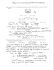### 36kW, 1000Vdc String Inverters for North America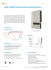### BT151(500R-650R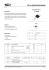### TS820-600T(800T)G - First Semiconductor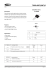### Linear Circuit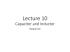### BT152-400R(600R,800R)G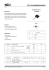### Misura della resistenza d`isolamento e della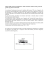### Formula Sheet for LSU Physics 2113, Third Exam, Fall ’14